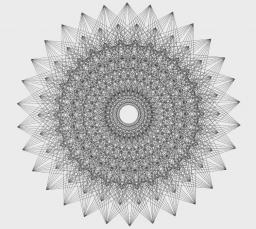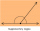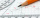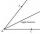# Neighbor angle

For 136° angle calculate size of adjacent angle on one side of a straight line.

x =  44 °

### Step-by-step explanation:

$x=18{0}^{\circ }-13{6}^{\circ }=44\text{°}$Did you find an error or inaccuracy? Feel free to write us. Thank you!## Related math problems and questions:

• RWYCalculate the opposite direction of the runway 13. Runways are named by a number between 01 and 36, which is generally one tenth of the azimuth of the runway's heading in degrees: a runway numbered 09 points east (90°), runway 18 is south (180°), runway 2
• TrianglesFind out whether given sizes of the angles can be interior angles of a triangle: a) 23°10',84°30',72°20' b) 90°,41°33',48°37' c) 14°51',90°,75°49' d) 58°58',59°59',60°3'
• MO Z7–I–6 2021In the triangle ABC, point D lies on the AC side and point E on the BC side. The sizes of the angles ABD, BAE, CAE and CBD are 30°, 60°, 20° and 30°, respectively. Find the size of the AED angle.
• Internal anglesThe ABCD is an isosceles trapezoid, which holds: |AB| = 2 |BC| = 2 |CD| = 2 |DA|: On its side BC is a K point such that |BK| = 2 |KC|, on its side CD is the point L such that |CL| = 2 |LD|, and on its side DA the point M is such that | DM | = 2 |MA|. Dete
• Inner anglesThe inner angles of the triangle are 30°, 45° and 105° and its longest side is 10 cm. Calculate the length of the shortest side, write the result in cm up to two decimal places.
• Supplementary angles(a + 30)° and (2a)° are the measure of two supplementary angles. Find the value of 'a'.
• Outer anglesThe outer angle of the triangle ABC at the A vertex is 71°40 ' outer angle at the vertex B is 136°50'. What size has the inner triangle angle at the vertex C?Convert magnitude of the angle α = 136°18'10" to radians:
• ConcertOn a Concert were sold 150 tickets for CZK 360, 235 tickets for 240 CZK and 412 for 180 CZK. How much was the total revenues for tickets?
• The sum 11The sum of the measures of two angles is 89°. One angle has a measure of 64°. What is the measure in degrees of the second angle?A ship travels 84 km on a bearing of 17° and then travels on a bearing of 107° for 135 km. Find the distance of the end of the trip from the starting point, to the nearest kilometer.Draw angle |∠ ABC| = 130° and built its axis. What angle is between axis angle and arm of angle?Calculate u.v if |u| = 5, |v| = 2 and when angle between the vectors u, v is: a) 60° b) 45° c) 120°Two forces with magnitudes of 25 and 30 pounds act on an object at 10° and 100° angles. Find the direction and magnitude of the resultant force. Round to two decimal places in all intermediate steps and your final answer.One angle of a rhombus is 136°, and the shorter diagonal is 8 cm long. Find the length of the longer diagonal and the side of the rhombus.Find all multiples of 10 that are larger than 136 and smaller than 214.The triangle is one outer angle 158°54' and one internal angle 148°. Calculate the other internal angles of a triangle.